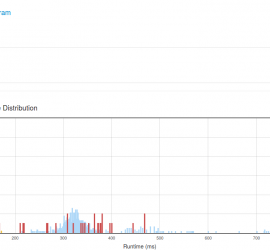# Daily Archives: January 24, 2015

## [leetcode] Largest Rectangle in Histogram

Largest Rectangle in Histogram Given n non-negative integers representing the histogram’s bar height where the width of each bar is 1, find the area of largest rectangle in the histogram. Above is a histogram where width of each bar is 1, given height = [2,1,5,6,2,3]. The largest rectangle is shown […]